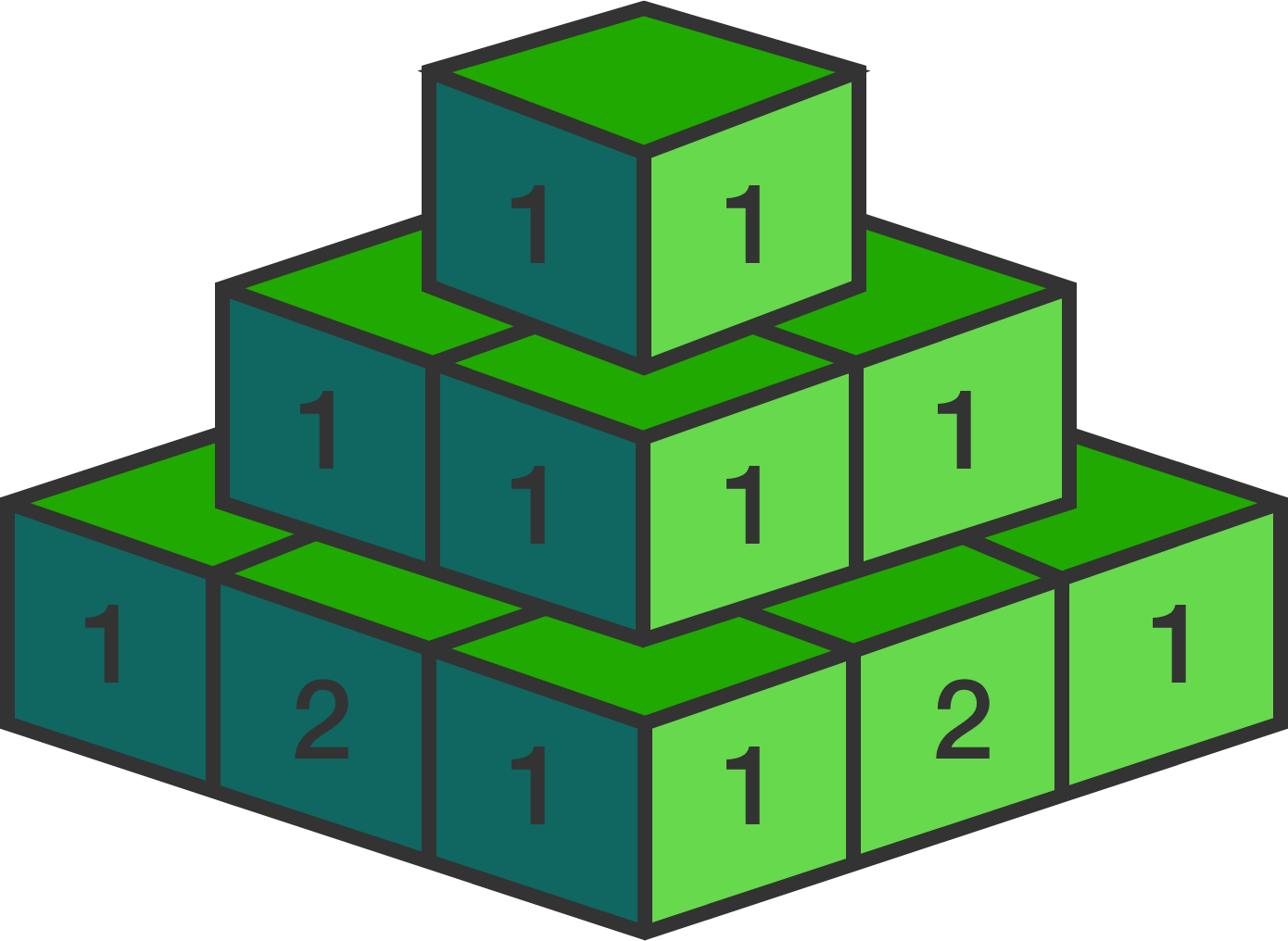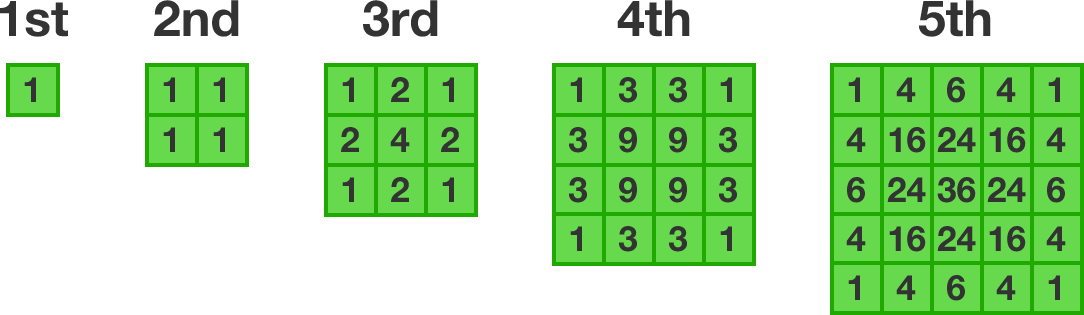# Pascal's Pyramid

Probability Level 2

Imagine a pyramid made out of blocks, where the first layer has 1 block, the second layer is a $2\times 2$ square made up of 4 blocks, the third layer is a $3\times 3$ square made up of 9 blocks, and so on, so that each $n^\text{th}$ layer is a square made from $n^2$ blocks.Each block has a number written on it. The top block has the number 1 written on it, but every other block has the sum of all the blocks that touch its top side written on it. The first five layers are as follows:What number would be written on the block that is in the $3^\text{rd}$ column and $4^\text{th}$ row of the $100^\text{th}$ layer?

Note: This problem does not require a programming solution, although you may want to use a calculator for the last step!

×